# NCERT class 7 chapter 6 try these• 2
It is in the Maths subject?
• -3
Which page try these
• -4
hey all try these you want
• 1
Do you need all
• 1
can you tell page no and sum

• 6
Hey all try do this question
• -5• -1
x=40 degree as base angles are equal
• 3
Yes need try theses answers of chapter 6.class 7
• 10
Yes i need all
• 6
I need try these answers from page 123
• 3
Try THESe
• -1
TRY THESE ch 6
• 0
WHAT IS INDIGESTON
• 0
Write the six elements (I.e.‚ the 3sides and the 3 angles) of angle ABC
• -3

Question 1:

In Δ ABC, X is the midpoint of BC.

AP is _____________

AX is _____________

Is BP = PC?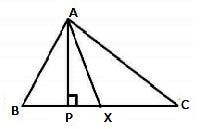Given:

BX = XC

Therefore, AP is altitude.

AX is a median.

No, BP ≠ PC as X is the midpoint of BC

Question 2:

Draw a sketch for the following:

(i) In Δ PQR, QX is a median

(ii) In Δ ABC, AB and AC are altitudes of a triangle.

(iii) In ABC, BP is an altitude in the exterior of a triangle.

(i) Here, QX is a median in ΔPQR and PX = XR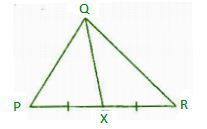(ii) Here, AB and AC are the altitudes of the ΔABC and CA ^ BA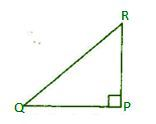(iii) BP is an altitude in the exterior of ΔABC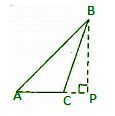Question 3:

Verify by drawing a diagram if the median and altitude of a isosceles triangle can be same.

Isosceles triangle means any two sides are same.

Take ΔPQR and draw the median when PQ= PR

PX is the median and altitude of the given triangle.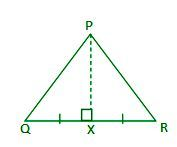• 3
I AM IN CLASS 6 ..SO :SORRY CANT TELL THE ANSWER .......
• -1